# Draw the graph of (i) x – 7y = – 42 (ii) x – 3y = 6 (iii) x – y + 1 = 0 (iv) 3x + 2y = 12 - Mathematics

Sum

Draw the graph of

(i) x – 7y = – 42

(ii) x – 3y = 6

(iii) x – y + 1 = 0

(iv) 3x + 2y = 12

#### Solution

(i) x – 7y = – 42

 x 0 7 14 y=(x+42)/7  6 7 8 Points A B C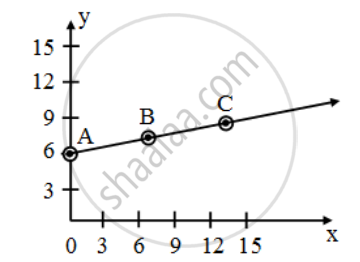(ii) x – 3y = 6

 x 6 12 18 y =(x-6)/3  0 2 4 Points D E F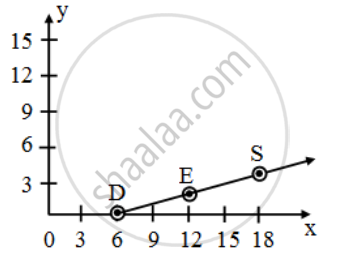(iii) x – y + 1 = 0

In tabular form

 x 0 4 y = x+1 1 5 Points A B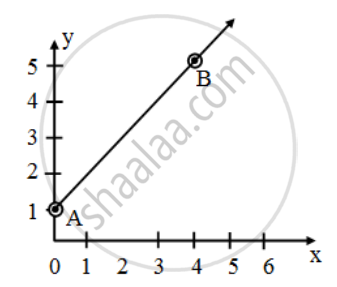(iv) 3x + 2y = 12

 x 0 2 y =(12-13x)/2  6 3 Point C D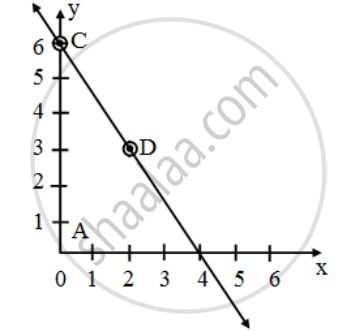Note:*
(i) The graph of any linear equation is a line and every solution of equations lies on the graph of that equation.
(ii) If a point (a, b) is not on the line then this point is not a solution of given equation.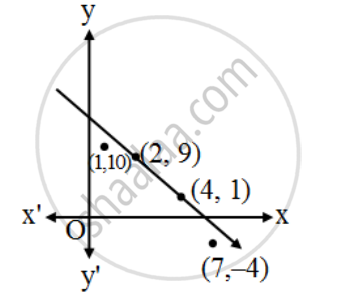∵ (2, 9) and (4, 1) are on the line

∴ These two points are solution of given equation

But (1, 10) and (7, –4) are not on the line so these two are not solutions.

Concept: Pair of Linear Equations in Two Variables
Is there an error in this question or solution?